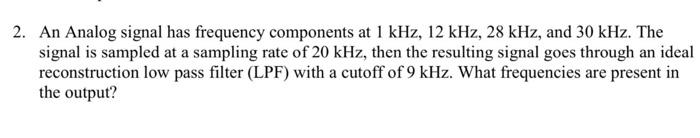Home / Expert Answers / Electrical Engineering / 2-an-analog-signal-has-frequency-components-at-1-mathrm-khz-12-mathrm-khz-28-mathrm-khz-pa675

# (Solved): 2. An Analog signal has frequency components at $$1 \mathrm{kHz}, 12 \mathrm{kHz}, 28 \mathrm{kHz} ...2. An Analog signal has frequency components at \( 1 \mathrm{kHz}, 12 \mathrm{kHz}, 28 \mathrm{kHz}$$, and $$30 \mathrm{kHz}$$. The signal is sampled at a sampling rate of $$20 \mathrm{kHz}$$, then the resulting signal goes through an ideal reconstruction low pass filter (LPF) with a cutoff of $$9 \mathrm{kHz}$$. What frequencies are present in the output?

We have an Answer from Expert